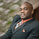1643 visitas
1643
(i) Arrow represent trade setup

(ii) Circle represent triggering of the trade
--------------------------------------------------------------------
1. Where to place stop loss?
2. Where to exit the trade?
3. How to protect gains in your trade?
4. How to apply money management rule?
5. How to trade gap opening?
```//  By SIMPLE TRADING TECHNIQUES

//   	Arrow represent trade setup
//   	Circle represent triggering of the trade
//--------------------------------------------------------------------

study(title="STT ENGULFING CANDLESTICK STRATEGY",overlay = true, shorttitle="STT ECS")
src = close, len = input(8, minval=1, title="Length")
up = rma(max(change(src), 0), len)
down = rma(-min(change(src), 0), len)
rsi = down == 0 ? 100 : up == 0 ? 0 : 100 - (100 / (1 + up / down))

//coloring method below

src1 = close, len1 = input(50, minval=1, title="UpLevel")
src2 = close, len2 = input(50, minval=1, title="DownLevel")
isup() => rsi > len1
isdown() => rsi < len2
barcolor(isup() ? green : isdown() ? red : na )

out = sma(close, 50)
data = rsi > len1 ? open > close ? close > open ? close >= open ? close >= open ? close - open > open - close ? high > out : na : na : na : na : na : na
data1 = rsi < len2 ? close > open ? open > close ? open >= close ? open >= close ? open - close > close - open ? low < out : na : na : na : na : na : na

plotchar(data, char='↑',location=location.belowbar, color=lime, text="ECS Buy")
plotchar(data1, char='↓', location=location.abovebar, color=red, text="ECS Sell")

tiggerlongcandle = (data == 1) and (high > high) ? 1 : 0
tiggershortcandle = (data1 == 1) and (low < low) ? 1 : 0
plotshape(tiggerlongcandle ? tiggerlongcandle : na, title="Triggered Long",style=shape.circle, location=location.belowbar, color=green, transp=0, offset=0, text="ECS")
plotshape(tiggershortcandle ? tiggershortcandle : na, title="Triggered Short",style=shape.circle, location=location.abovebar, color=red, transp=0, offset=0, text="ECS")

plot (out, color = black, linewidth = 3, title = "Trend - Long Term")
```mcommorato
@mcommorato, Yes it's the 50-period simple moving average.
Respondershani_1694
i don't understand the question, can reword it please.
Respondershani_1694
this script is not for the pullback candlestick strategy, it is for another strategy that i am working on. in order the get the script for the pullback candlestick strategy search for it in the indicators public library.
Respondershani_1694
that is a trade setup (signal) but the trade was not trigger. ARROW represent trade setup while CIRCLE represent triggering of the trade
Responder